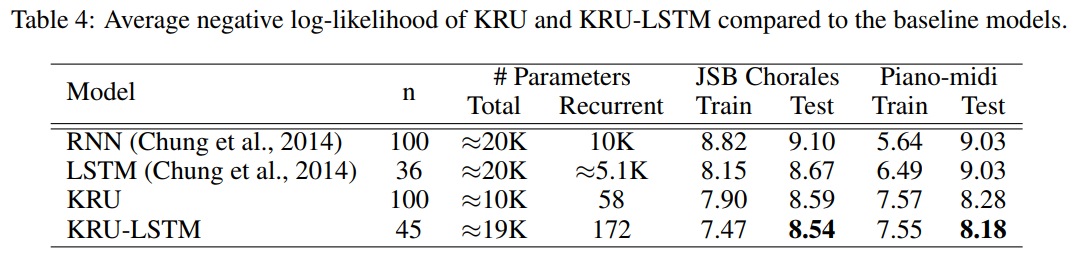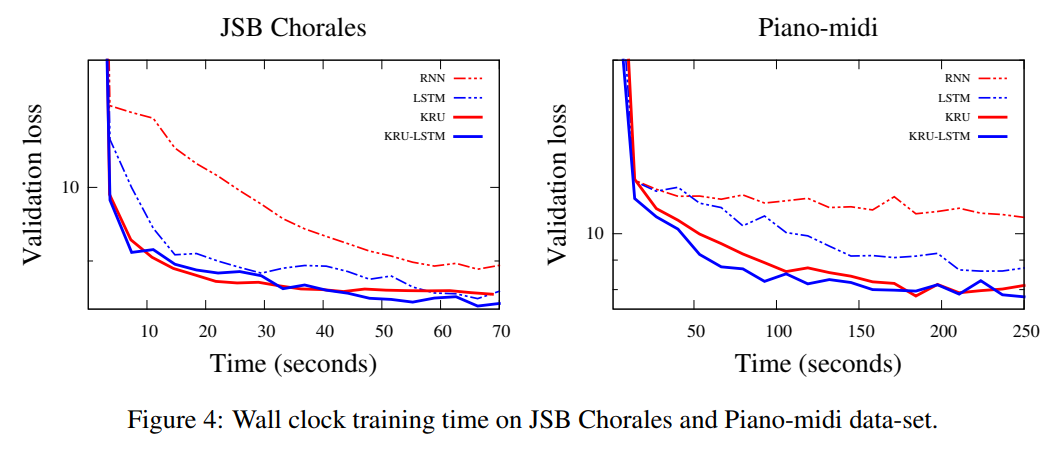## Summary

### Goals

• Better weight efficiency
• Better conditioning of the recurrent weight matrix (prevent vanishing/exploding gradients)

#### Reminder: Vanilla RNN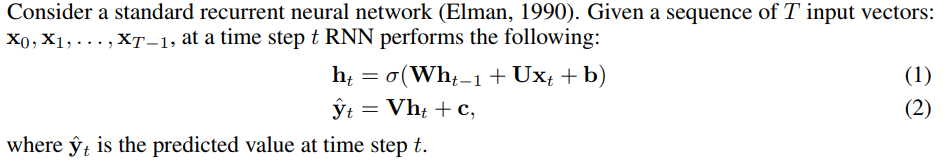### Core idea

Factorize the NxN recurrent matrix using a Kronecker factorization. This allows some flexibility in the actual number of parameters through the size of the factors.

For example, using 2x2 factors, there are $$O(\log(N))$$ parameters, whereas it can go up to $$O(N^2)$$ parameters when using a single NxN factor (the original RNN description).

The vanishing and exploding gradient problem is tackled through a soft unitary constraint, which is easier to apply when using small factors.

NOTE: This soft constraint is not needed when using an LSTM, since the gating mechanism is already designed to prevent exploding/vanishing gradients.

#### Reminder: Kronecker product#### Reminder: Unitary matrix

In real space, a unitary matrix is simply an orthogonoal matrix, i.e. its transpose is also its inverse:

$Q^T Q = QQ^t = I \Leftrightarrow Q^T = Q^{-1}$

In complex space, a matrix is unitary if its conjugate transpose is also its inverse:

$U^* U = UU^* = I$

(Remember, the conjugate transpose is the transpose matrix where the imaginary parts have their sign reversed.)

### Factorization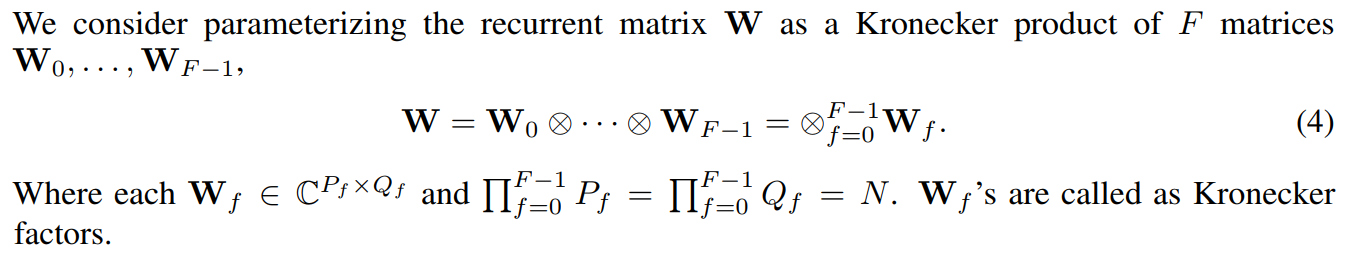If factors are 2 x 2, then there are $$\log_2 N$$ factors, the number of parameters is $$8 \log_2 N$$ (when using complex factors), and the time complexity of the hidden state computation is $$O(N \log_2 N)$$.

If factors are N x N, then there is a single N x X factor and the standard RNN is recovered.

Thus, there is flexibility between computational budget and statistical performance.

### Soft unitary constraint

A regularization term is simply added to the loss :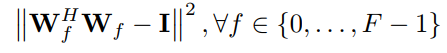If all the factors are unitary, then the full matrix W is unitary, and if all factors are approximately unitary, then W is also approximately unitary. This new loss term introduces the usual strength hyperparameter like any other regularization term.

### Using complex matrices

Imposing a unitary constraint on a real matrix means that the space it can cover is disconnected (because its determinant is either 1 or -1). Using a complex space means that the unitary set is now connected and continuous, and is more easily optimized.

## Experiments

NOTE: The authors implemented their own custom CUDA kernels for fast Kronecker operations in C++ (inexistent in Theano, Tensorflow and Pytorch)

### Copy-memory problem (sequence to sequence)

Input : $$[x_0, x_1, ..., x_9, \text{blank} \times T-1, \text{delimiter}, \text{blank} \times 10]$$

Target : $$[\text{blank} \times T+10, x_0, x_1, ..., x_9]$$

NOTE: Expected cross-entropy for a memory-less strategy is 0.03 for T=1000 and 0.015 for T=2000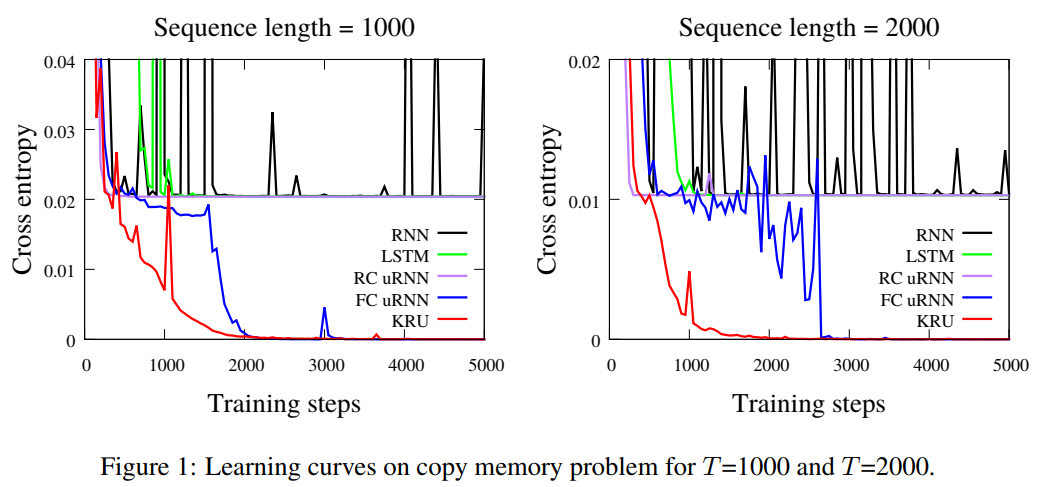Input : Two sequences (first a sequence of random numbers, then a sequence of equal size, filled with zeros but containing two 1s at random places)

Target : Predict the sum of the numbers in the 1st sequence at the positions defined the ones in the 2nd sequence.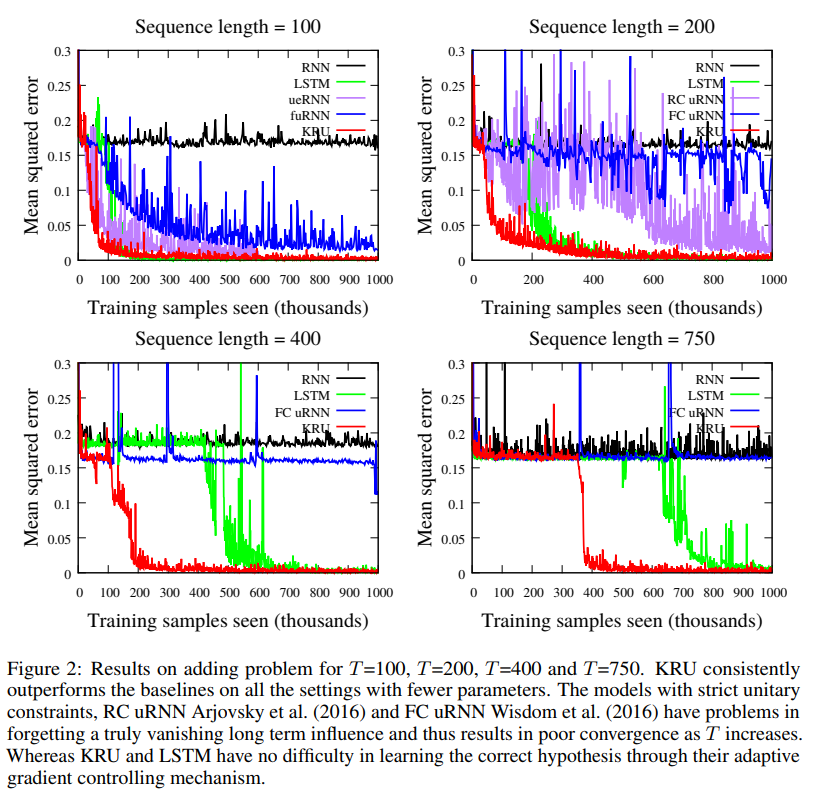### Pixel-by-pixel MNIST classification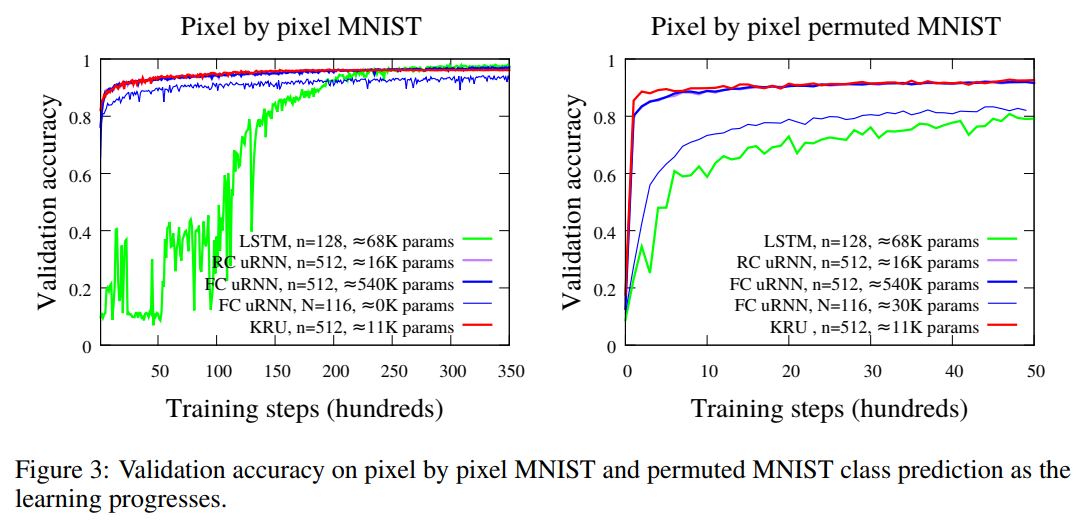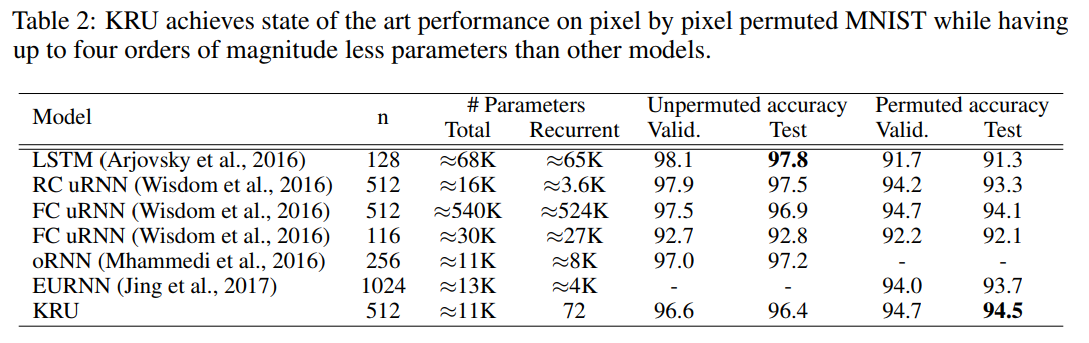### Penn-Treebank character-level modeling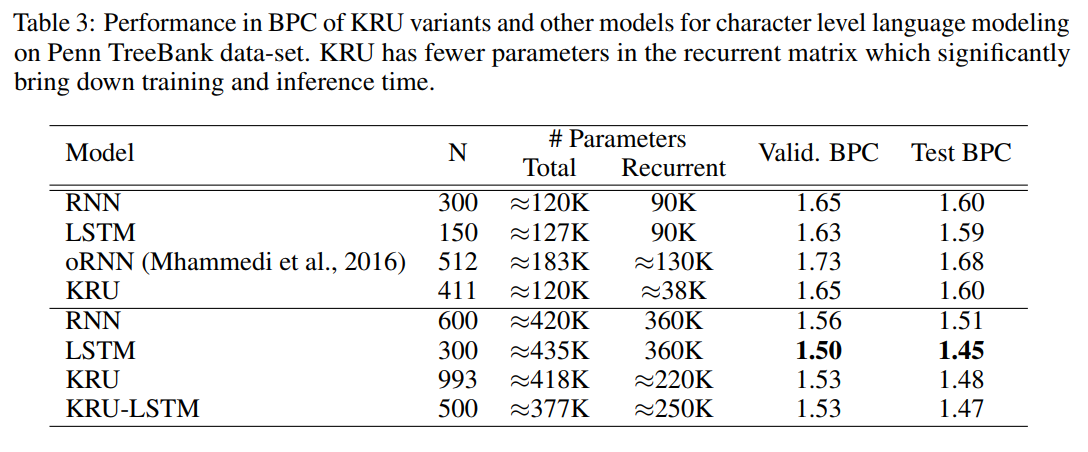### Music modeling